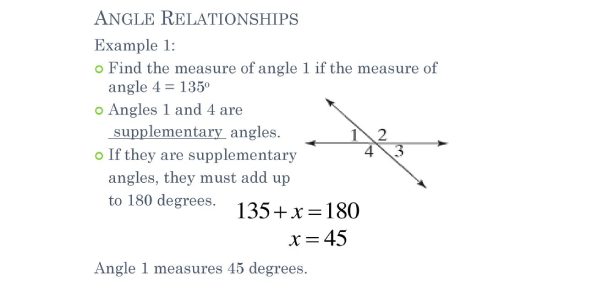# Angle Relationships Quiz Questions

5 Questions | Total Attempts: 1922Settings• 1.
Identify the angle relationship between the two angles shown.
• A.

Vertical Angles

• B.

• C.

• D.

Congruent Angles

• 2.
Identify the angle relationship of these two angles.
• A.

• B.

Supplementary Angles

• C.

Vertical Angles

• D.

• 3.
Another name for supplementary angles is ___________________________.
• A.

• B.

Vertical Angles

• C.

Congruent Angles

• D.

Linear Pair

• 4.
Complementary angles are ALWAYS adjacent angles.
• A.

True

• B.

False

• 5.
What is the measure of angle A?
• A.

28 degrees

• B.

62 degrees

• C.

118 degrees

• D.

45 degrees

Related TopicsBack to top